Next: Type I Superstrings Up: Perturbative String and Superstring Previous: Interacting Strings and the

## Superstrings

In bosonic string theory there are two bold problems. The first one is the presence of tachyons in the spectrum. The second one is that there are no spacetime fermions. Here is where superstrings come to the rescue. A superstring is described, despite of the usual bosonic fields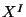, by fermionic fields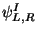on the worldsheet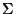. Which satisfy anticommutation rules and where the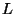and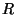denote the left and right worldsheet chirality respectively. The action for the superstring is given by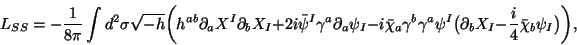(25)

where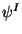and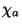are the superpartners ofand the tetrad field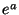respectively. In the superconformal gauge (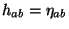and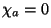) and in light-cone coordinates it can be reduced to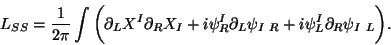(26)

In analogy to the bosonic case, the local dynamics of the worldsheet metric is manifestly conformal anomaly free at the quantum level if the critical spacetime dimension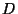is 10. Thus the string oscillates in the 8 transverse dimensions. The action (25) is invariant under: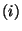worldsheet supersymmetry,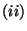Weyl transformations,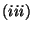super-Weyl transformations,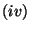Poincaré transformations and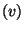Worldsheet reparametrizations. The equation of motion for the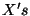fields is the same that in the bosonic case (Laplace equation) and whose general solution is given by Eqs. (5) or (6). Equation of motion for the fermionic field is the Dirac equation in two dimensions. Constraints here are more involved and they are called the super-Virasoro constraints. However in the light-cone gauge, everything simplifies and the transverse coordinates (eight coordinates) become the bosonic physical degrees of freedom together with their corresponding supersymmetric partners. Analogously to the bosonic case, massless states of the spectrum come into representations of the little group SO(8) which is a subgroup of SO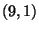, while that the massive states lie into representations of the little group SO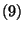. For the closed string there are two possibilities for the boundary conditions of fermions:periodic boundary conditions (Ramond (R) sector)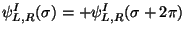andanti-periodic boundary conditions (Neveu-Schwarz (NS) sector)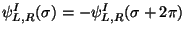. Solutions of Dirac equation satisfying these boundary conditions are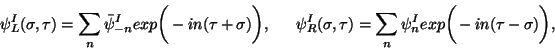(27)

where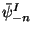and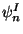are fermionic modes of left and right movers respectively. In the case of the fermions in the R sector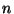is integer and it is semi-integer in the NS sector. The quantization of the superstring come from the promotion of the fieldsandto operators whose oscillator variables are operators satisfying the relations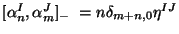and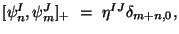where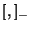and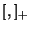stand for commutator and anti-commutator respectively. The zero modes of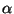are diagonal in the Fock space and its eigenvalue can be identified with its momentum. For the NS sector there is no fermionic zero modes but they can exist for the R sector and they satisfy a Clifford algebra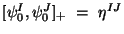. The Hamiltonian for the closed superstring is given by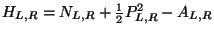. For the NS sector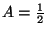, while for the R sector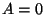. The mass is given by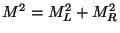with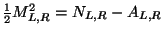. There are five consistent superstring theories: Type IIA, IIB, Type I, SO(32) and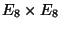heterotic strings, represented by HO and HE respectively. In what follows of this section we briefly describe the spectrum in each one of them. truecm Type II Superstring Theories In this case the theory consist of closed strings only. They are theories with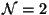spacetime supersymmetry. There are 8 scalar fields (representing the 8 transverse coordinates to the string) and one Weyl-Majorana spinor. There are 8 left-moving and 8 right-moving fermions. In the NS sector there is still a tachyon in the ground state. But in the supersymmetric case this problem can be solved through the introduction of the called GSO projection. This projection eliminates the tachyon in the NS sector and it acts in the R sector as a ten-dimensional spacetime chirality operator. That means that the application of the GSO projection operator defines the chirality of a massless spinor in the R sector. Thus from the left and right moving sectors, one can construct states in four different sectors:NS-NS,NS-R,R-NS andR-R. Taking into account the two types of chiralityandone has two possibilities: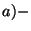The GSO projections on the left and right fermions produce different chirality in the ground state of the R sector (Type IIA).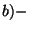GSO projection are equal in left and right sectors and the ground states in the R sector, have the same chirality (Type IIB). Thus the spectrum for the Type IIA and IIB superstring theories is:
• Type IIA The NS-NS sector has a symmetric tensor field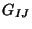(spacetime metric), an antisymmetric tensor field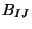and a scalar field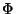(dilaton). In the R-R sector there is a vector field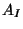associated with a 1-form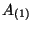(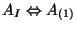) and a rank 3 totally antisymmetric tensor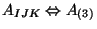and by Hodge duality in ten dimensions also we have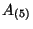,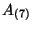and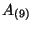. In general the R-R sector consist of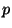-forms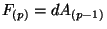(where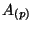are called RR fields) on the ten-dimensional spacetime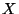witheven i.e.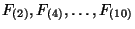. In the NS-R and R-NS sectors we have two gravitinos with opposite chirality and the supersymmetric partners of the mentioned bosonic fields.
• Type IIB In the NS-NS sector Type IIB theory has exactly the same spectrum that of Type IIA theory. On the R-R sector it has a scalar field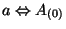(the axion field), an antisymmetric tensor field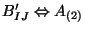and a rank 4 totally antisymmetric tensor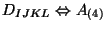whose field strength is self-dual i.e.,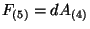with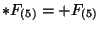. Similar than for the case of Type IIA theory one has also the Hodge dual fields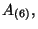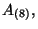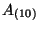. In general, RR fields in Type IIB theory are given by-formson the spacetimewithodd i.e.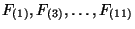. The NS-R and R-NS sectors do contain two gravitinos with the same chirality and the corresponding fermionic matter.
truecmNext: Type I Superstrings Up: Perturbative String and Superstring Previous: Interacting Strings and the
root 2001-01-15